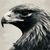# Looping through XYZ coordinats

228
4
04-25-2022 01:34 PMby
New Contributor

Hi, I working on a road dataset and found all the roads that intersect, like the to roads below:I want to investigate if the z-value from the two roads are the same. I used arcpy.FeatureVerticesToPoints_management the get the XYZ values from each road, and load them into two python list, like in the example below:

The first think I want to do, is to check whatever the roads share vertices (Same XY value) and if the do, whats the difference in their z-value.

I tried with this function:

def compareLineVertiex(list_orginid,list_destid):
sameXY = []
for (x,y,z) in list_orginid:
xy = x,y
if xy in list_destid:

sameXY.append(xyz)
return sameXY

I only managed to compare their XY values. I am missing the z-value (which will be difference from the two list)

1 Solution

Accepted SolutionsOccasional Contributor III

Maybe a little long but how's this

``````def compare_vertices(linea, lineb):
matches = []
for i_idx, a in enumerate(linea):
for b_idx, b in enumerate(lineb):
if [a, a] == [b, b]:
if a - b > 0:
zdiff = a - b
else:
zdiff = b - a
matches.append({
"Point A": {
"XYZ": a,
"Index": a_idx,
},
"Point B": {
"XYZ": b,
"Index": b_idx,
},
"Z diff": zdiff
})
return matches

road1 = [(1, 2, 3), (3, 4, 5), (4, 6, 8)]
road2 = [(3, 4, 6), (1, 2, 4), (4, 5, 3)]

for i in x:
print(i)

{'Point A': {'XYZ': (1, 2, 3), 'Index': 0}, 'Point B': {'XYZ': (1, 2, 4), 'Index': 1}, 'Z diff': 1}
{'Point A': {'XYZ': (3, 4, 5), 'Index': 1}, 'Point B': {'XYZ': (3, 4, 6), 'Index': 0}, 'Z diff': 1}``````

I only enumerated to know where the points are that match in the array, and returned as a dict because I'm fussy like that and want more info than less usually

4 RepliesOccasional Contributor III

Maybe a little long but how's this

``````def compare_vertices(linea, lineb):
matches = []
for i_idx, a in enumerate(linea):
for b_idx, b in enumerate(lineb):
if [a, a] == [b, b]:
if a - b > 0:
zdiff = a - b
else:
zdiff = b - a
matches.append({
"Point A": {
"XYZ": a,
"Index": a_idx,
},
"Point B": {
"XYZ": b,
"Index": b_idx,
},
"Z diff": zdiff
})
return matches

road1 = [(1, 2, 3), (3, 4, 5), (4, 6, 8)]
road2 = [(3, 4, 6), (1, 2, 4), (4, 5, 3)]

for i in x:
print(i)

{'Point A': {'XYZ': (1, 2, 3), 'Index': 0}, 'Point B': {'XYZ': (1, 2, 4), 'Index': 1}, 'Z diff': 1}
{'Point A': {'XYZ': (3, 4, 5), 'Index': 1}, 'Point B': {'XYZ': (3, 4, 6), 'Index': 0}, 'Z diff': 1}``````

I only enumerated to know where the points are that match in the array, and returned as a dict because I'm fussy like that and want more info than less usuallyby
New Contributor

Thanks 🙂 this well get the job done. I did a test and its working on the dataset.byMVP Esteemed Contributor

numpy anyone?

``````road1 = np.array([(1, 2, 3), (3, 4, 5), (4, 6, 8)])
road2 = np.array([(3, 4, 6), (1, 2, 4), (4, 5, 3)])

xyz = []
x, y, z1 = row
chk = (road2[:, :2][:, None] == row[:2]).all(-1)
if chk.any():
w = np.nonzero(chk)
vals = [x, y, z1, z2[-1]]
xyz.append(vals)
xyz1z2 = np.array(xyz)

xyz1z2
array([[1, 2, 3, 4],
[3, 4, 5, 6]])``````

To bring it back into Pro

``````dt = [('X', 'f8'), ('Y', 'f8'), ('Z1', 'f8'), ('Z2', 'f8')]

# -- do it the slow way
out = np.zeros(xyz1z2.shape, dtype=dt)
out['X'] = xyz1z2[:, 0]
out['Y'] = xyz1z2[:, 1]
out['Z1'] = xyz1z2[:, 2]
out['Z2'] = xyz1z2[:, 3]
out
array([(  1.00,   2.00,   3.00,   4.00), (  3.00,   4.00,   5.00,   6.00)],
dtype=[('X', '<f8'), ('Y', '<f8'), ('Z1', '<f8'), ('Z2', '<f8')])
# --- then
arcpy.da.NumPyArrayToTable(out, path_and_tbl_name)``````

... sort of retired...by
New Contributor

Thanks 🙂 I will try it out.